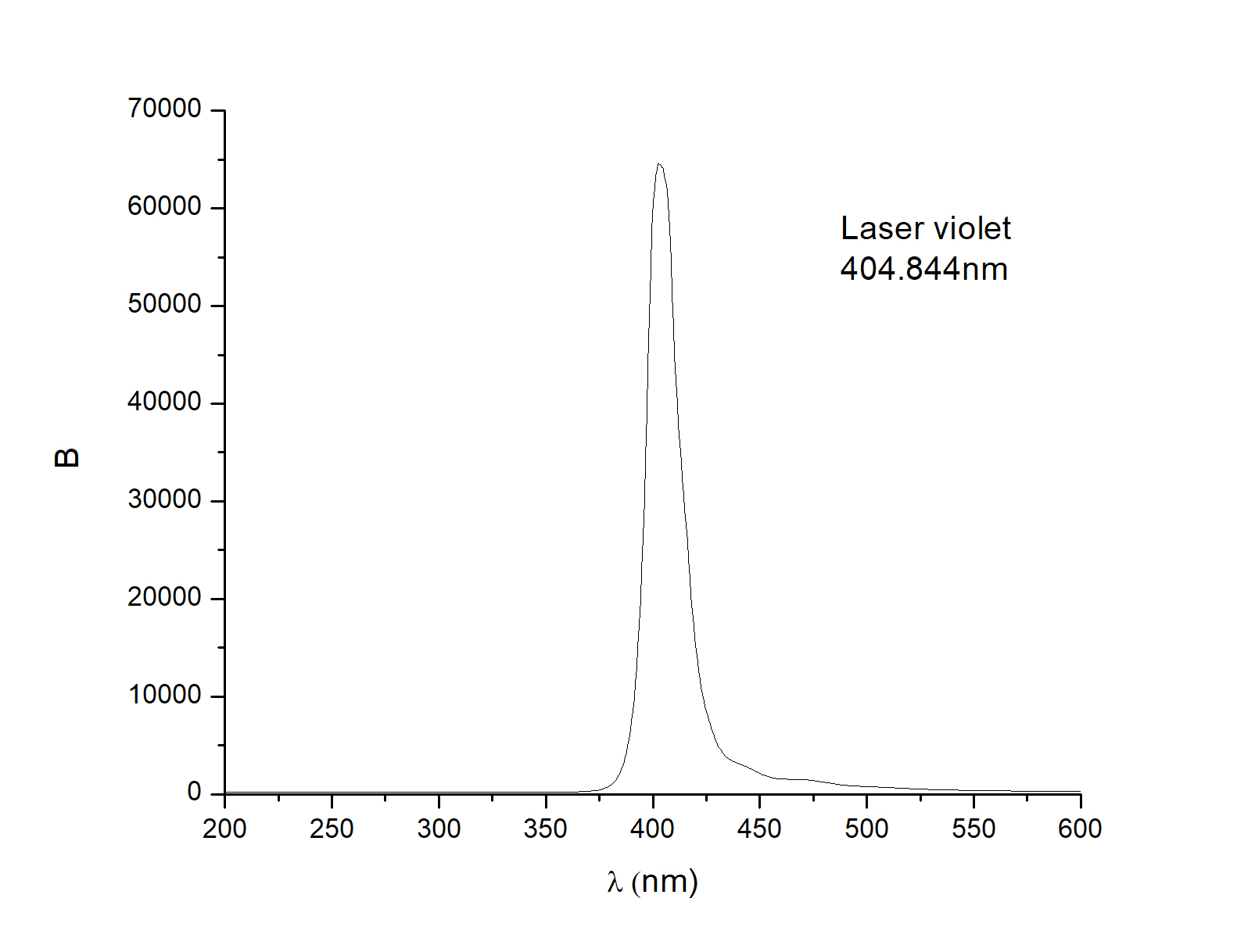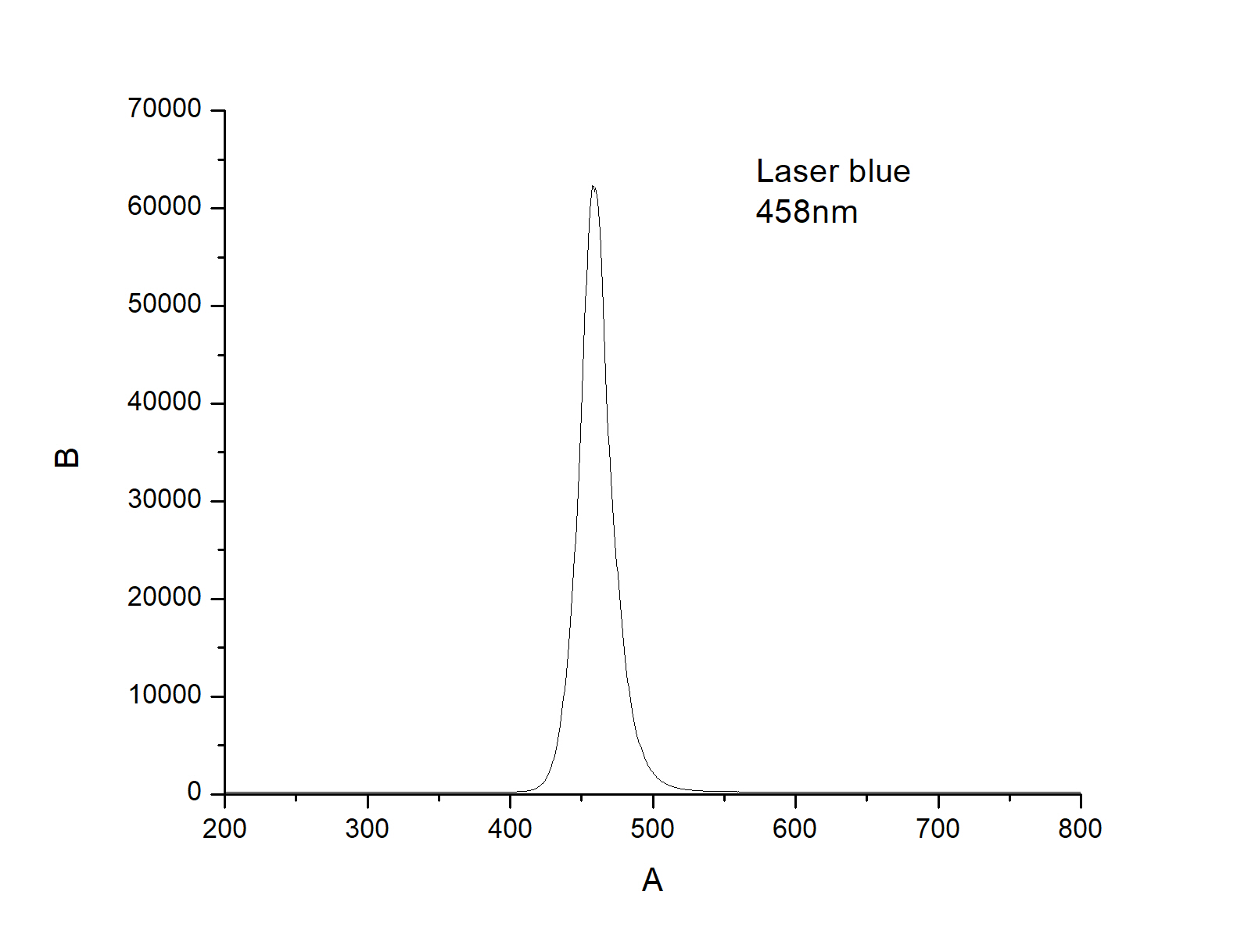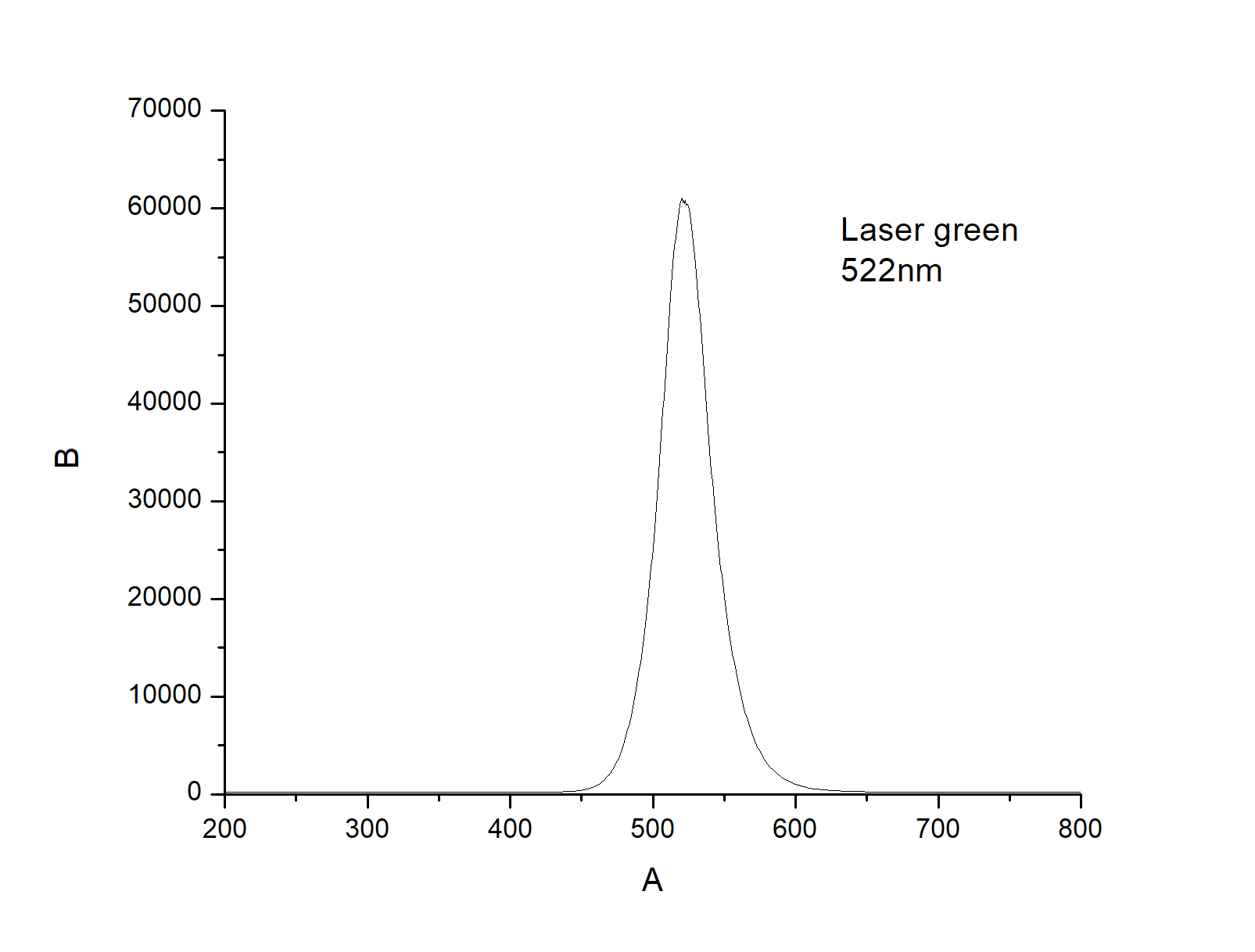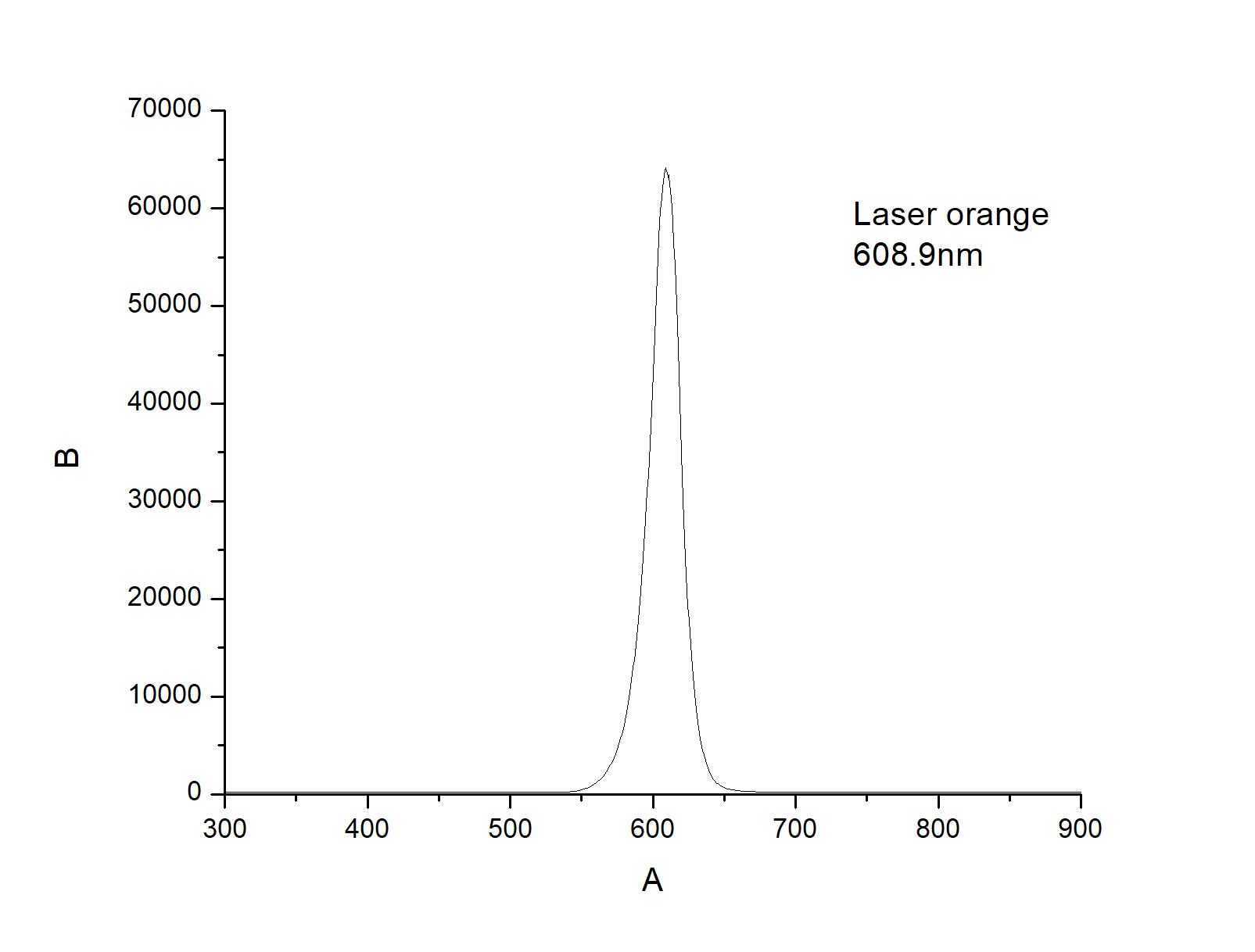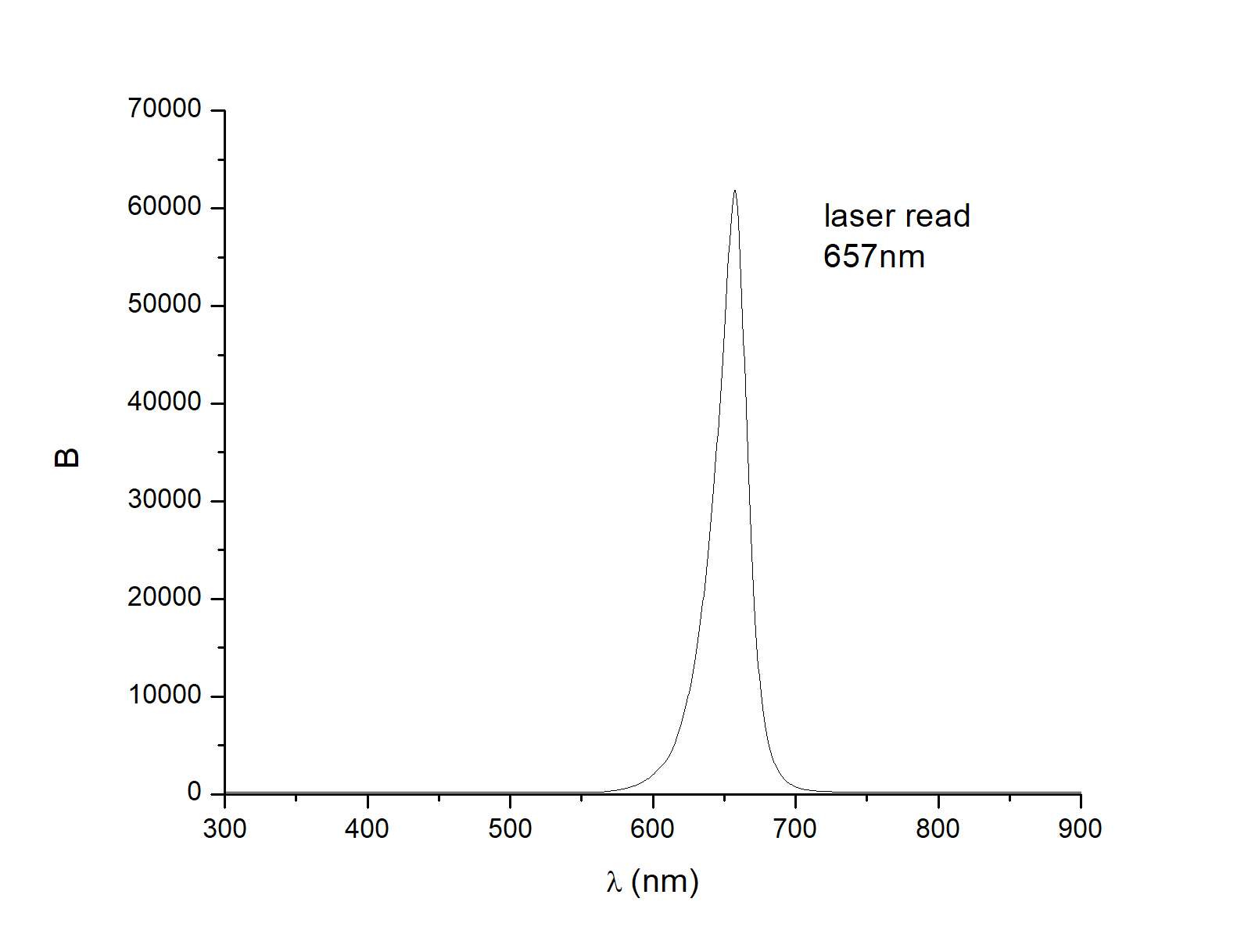# External Photoelectric Effect. Determine Planck's constant

\$700.00

Code Number: PTVL2018_Planck

Brand: AELAB

#### Purpose:

1. Observe and comment on external photoelectric effects with different wavelength light.
2. Investigate the saturation of photoelectric current Ic
3. Determine Planck's constant
- +

Product will be printed in English

Planck's constant experiment uses monochrome light as LEDs.

The wavelength of this monochromatic light is precisely measured by UV-VIS spectrophotometer from OceanOptic.

The test set did not use any amplifier to amplify the electrical signal, so the results were reliable.

This experiment including:

+ 5 LED lights: Red, Orange, green, blue and violet.

+ Multimeter

#### Object of experiment:

1. Observe and comment on external photoelectric effects with different wavelength light.
2. Investigate the saturation of photoelectric current Ic
3. Determine Planck's constant

#### 1. Observe and comment on external photoelectric effects with different wavelength light

Reverse switch is in Forward status: That means the positive pole of the power source will connect to the anode of the photocell, and the negative pole of the source will connect with the cathode

+ With RED light: When the voltage is set to 0 (0.007V), adjust the brightness of the brightest light. The Dot light of galvanometer does not change.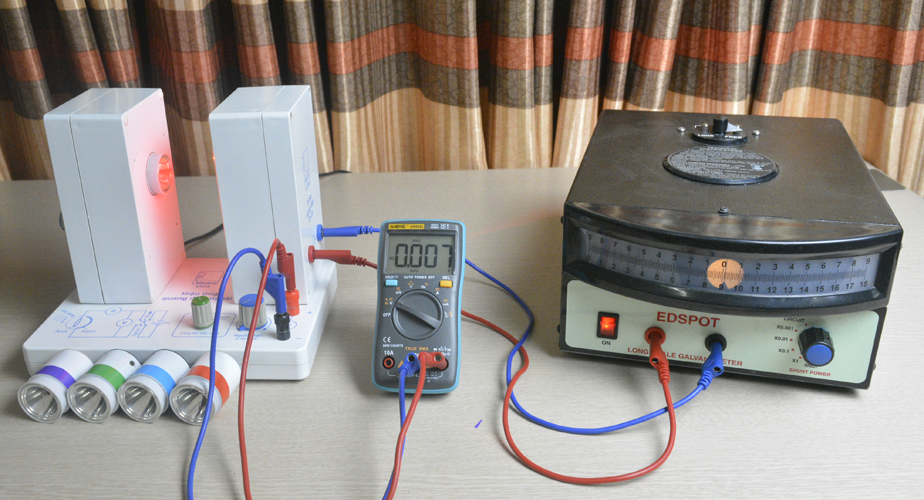Photoelectric effect experiment: Red light

When adjusting the maximum voltage (turn all the way to the right), the galvanometer indicator slightly deviates: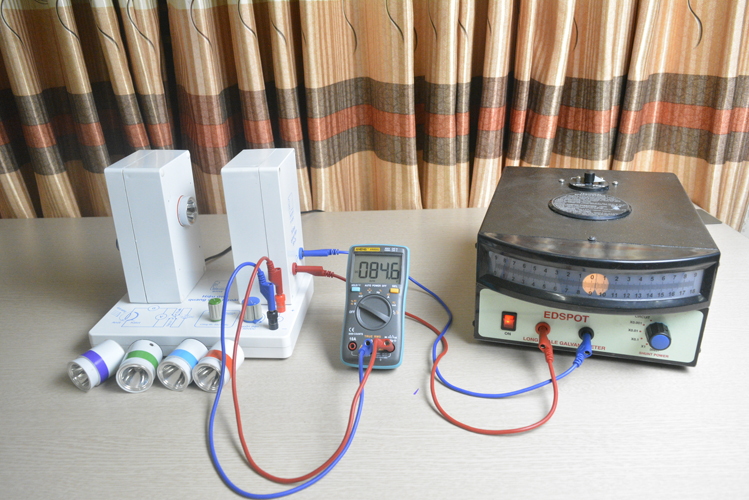Photoelectric effect experiment:: Indicator deviates slightly.

Hypothesis: The galvanometer needle is slightly skewed (3mm) with red light because we put a high voltage between the anodes and the cathode of the photocell

+ With orange light: we do the same as with red light: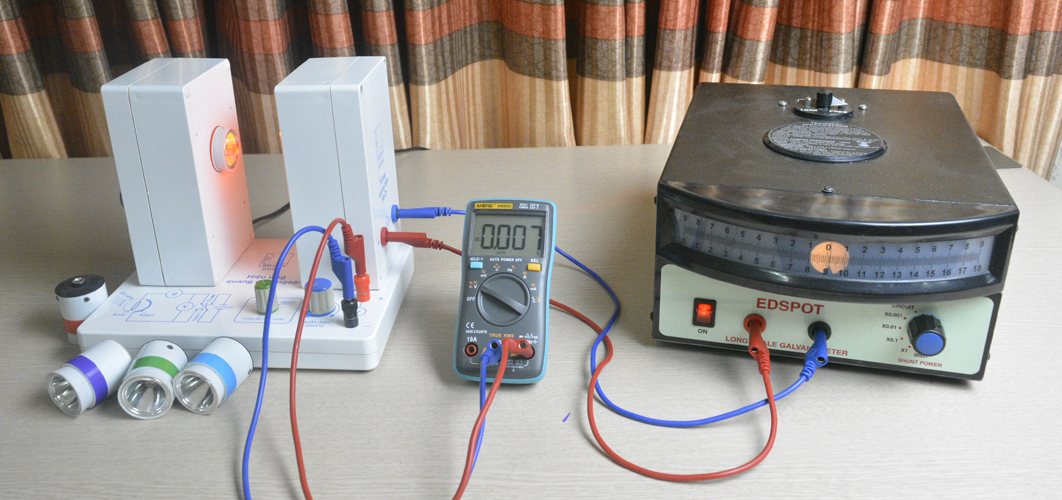Planck's constant experiment: Orange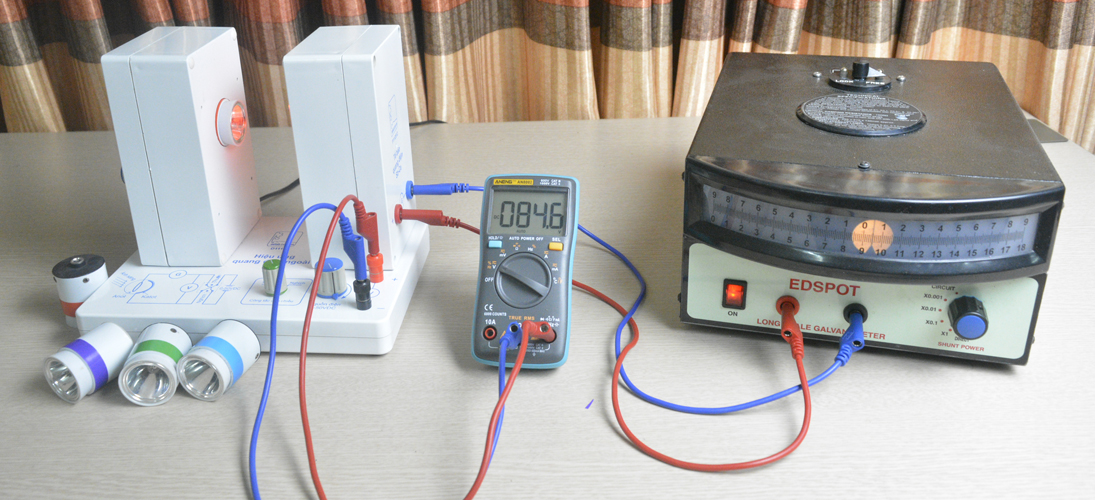Photoelectric effect experiment: Indicator deviates slightly.

Hypothesis: The galvanometer needle is slightly off (about 1mm) when the voltage is 0, When the maximum voltage is, the galvanometer needle deviates by 6mm.

We do the same with the green, blue and purple light. The following results:

Green light: When the potential is zero, the light of the galvanometer needle is 28mm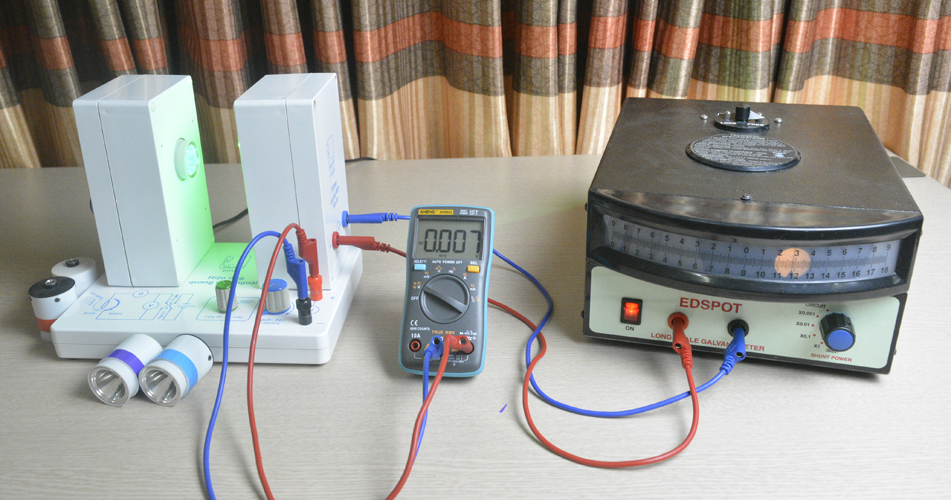Photoelectric effect experiment: Green light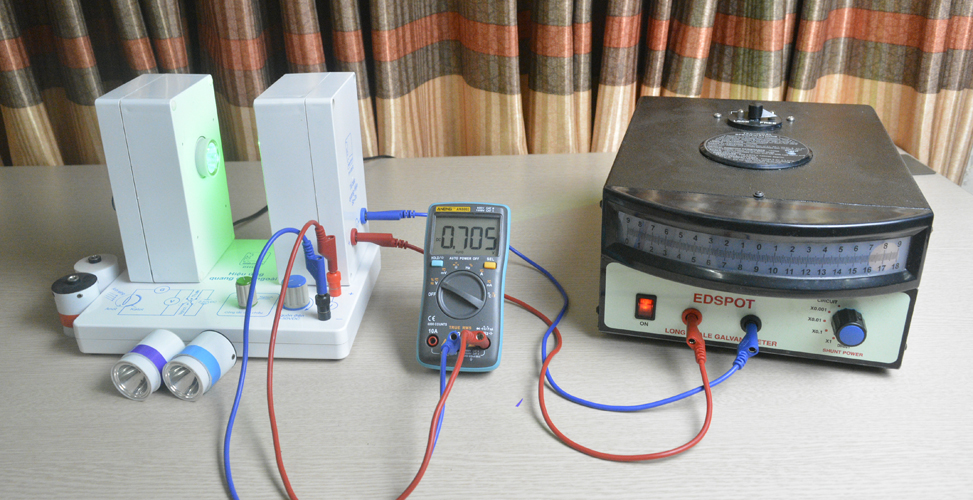Photoelectric effect experiment: Indicator deviates much

Blue light: When the voltage is zero, the brightest light on the galvanometer deviates from the scale of the galvanometer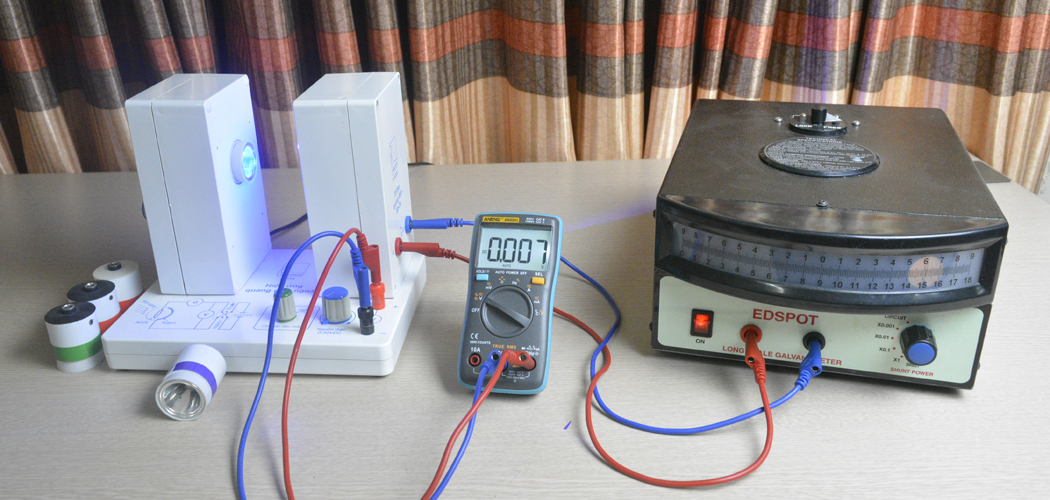Photoelectric effect experiment: Blue light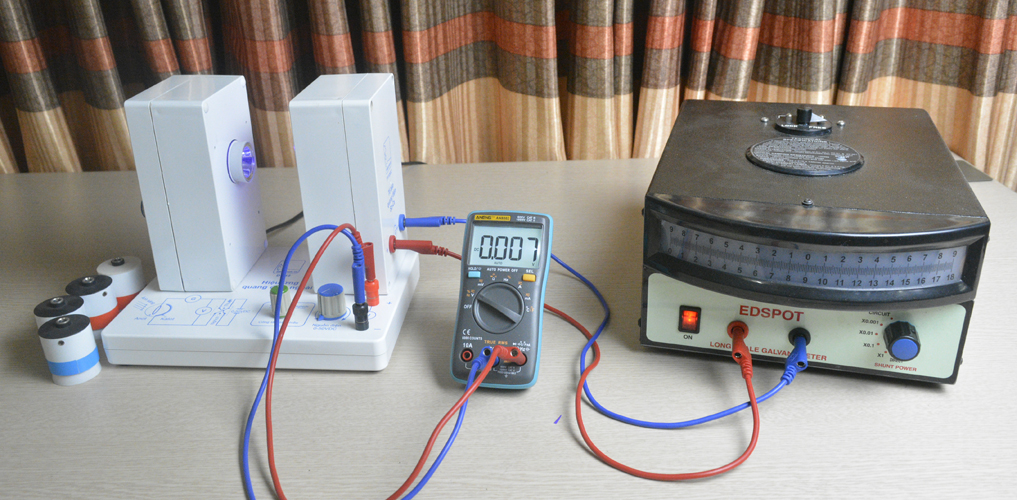Photoelectric effect experiment: Indicator deviates much

Violet light: When the potential is zero, the galvanometer deviates the most.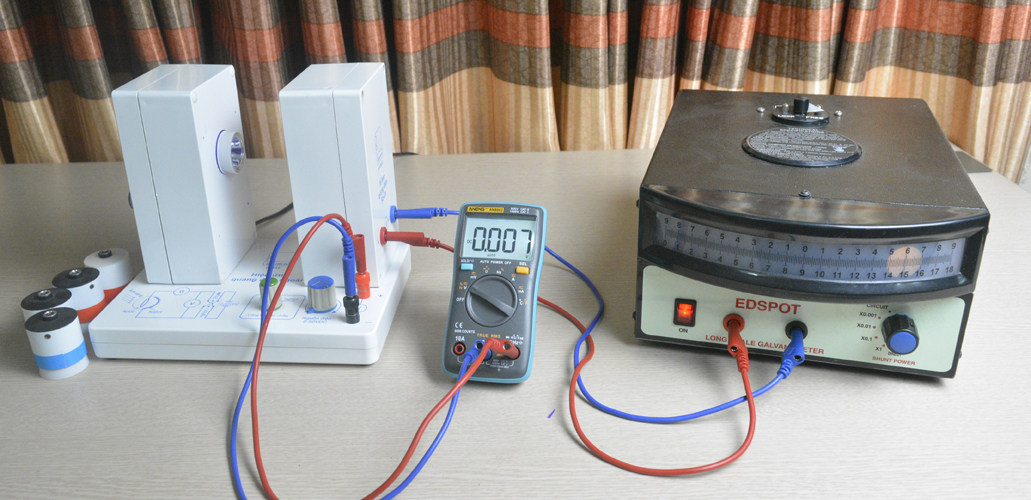Photoelectric effect experiment: Violet

Conclusion: For students

#### 2. Investigate the saturation of photoelectric current Ic

We do not publish the results of this task because it's simple.

#### 3. Determine Planck's constant

Reverse Switch is on Reverse status:At this time, the positive pole of the source will connect with the cathode and the negative pole of the source will connect to the anode of the photocell.

+ We do experiments with green light:

++ We increase the light so that the galvanometer needle is slightly off (about 3mm) when the voltage is zero.Planck's constant experiment: Green LED

++ We gently rotate the potentiometer knob so that the pointer of the galvanometer reaches zero (the initial position of the galvanometer). Then we slightly increased the brightness of the lamp as the galvanometer deviates slightly. Then continue adjusting the voltage very slightly so that the galvanometer reaches zero.

Up to a voltage that when the maximum brightness is increased, the galvanometer needle almost stable still and did not move. That is the stopping potential Us of green light.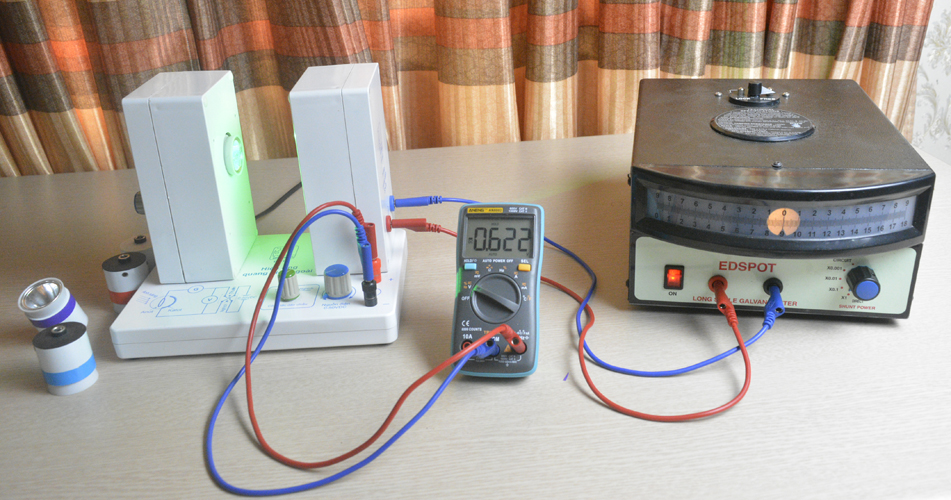Planck's constant experiment: Stopping Voltage

++ With green light we measure Usg = 0.622V

+ We do the same with the blue light: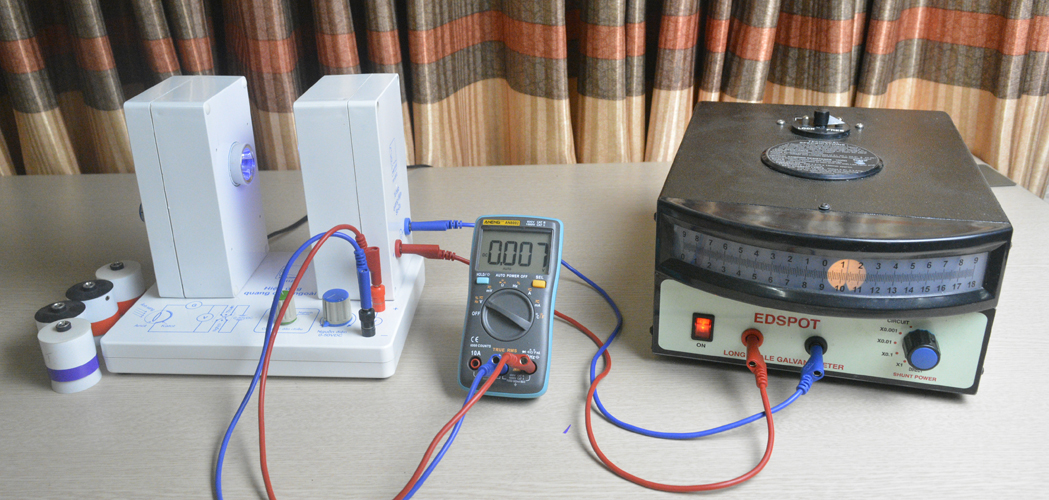Planck's constant experiment: Blue light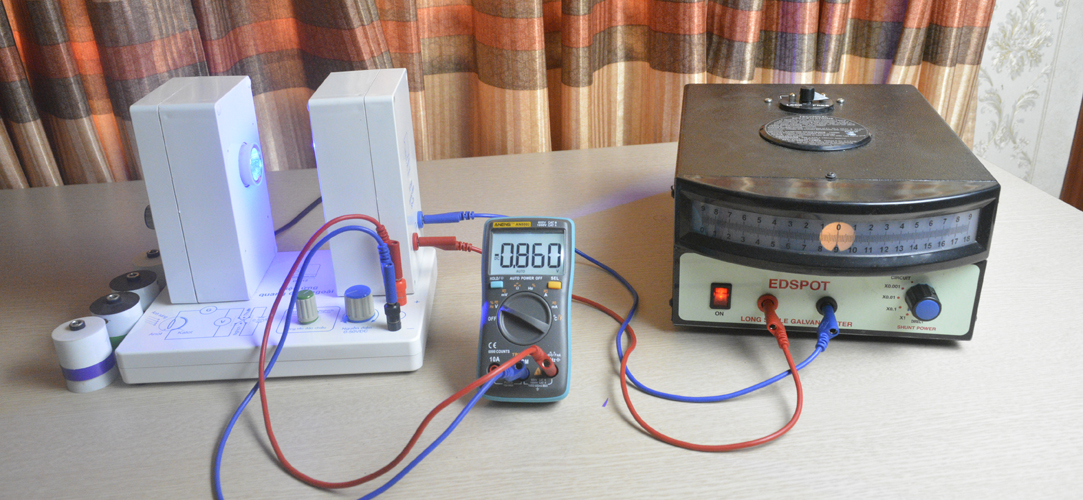Planck's constant experiment: Stopping Voltage

++ With green light we measure Usb = 0.860V

+ We do the same with violet light: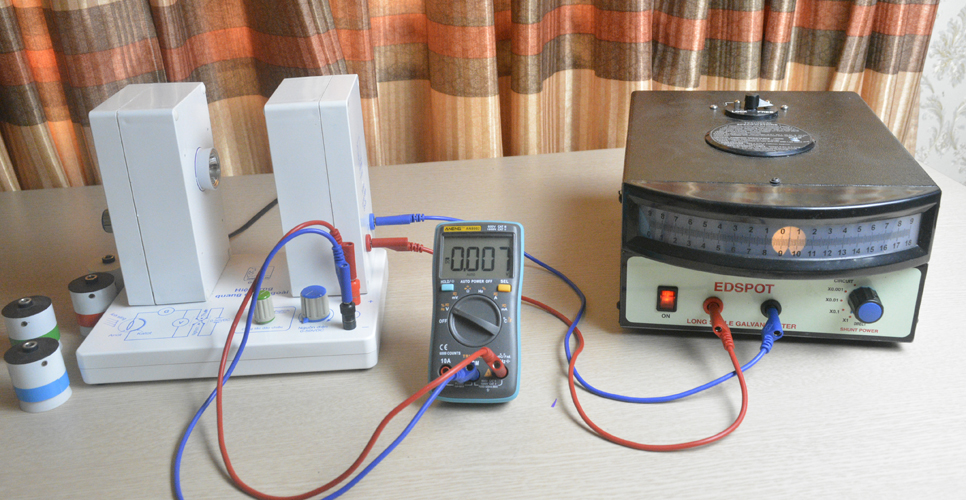Planck's constant experiment: Violet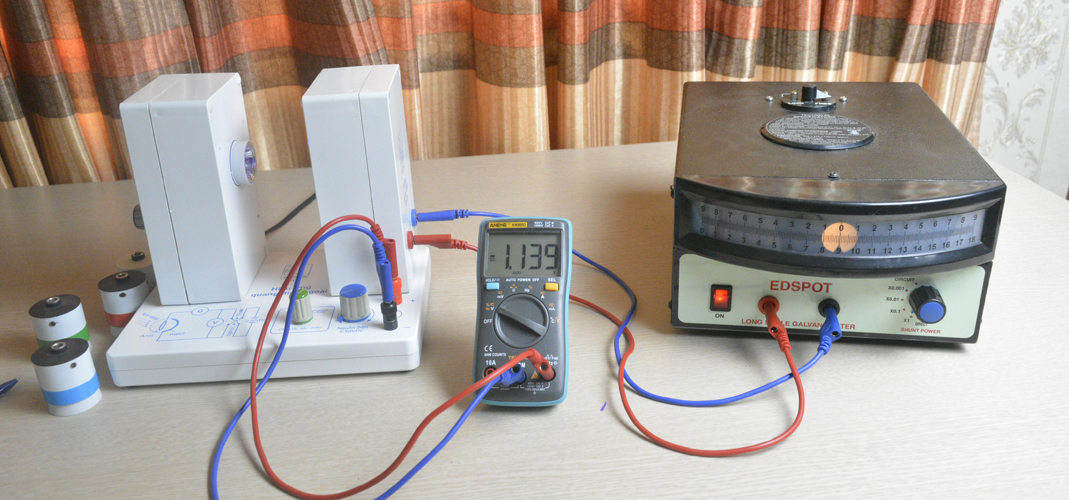Planck's constant experiment: Stopping Voltage

++ With violet light we can measure Usv = 1,139V

With the following formula, planck h can be calculated: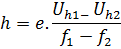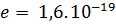f: is the frequency of light.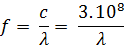So to determine h we need to know the wavelength λ of the green, blue and violet light and the stopping potential Us (measured above)

Light wavelength is measured by spectroscopy firm Oceanoptics - USA, we have the following data table:

 Light Wavelength λ Green 522nm Blue 458nm Violet 405nm

the frequency of light.:

 Light Tần số f (Hz) Green 575.10e-12 Blue 655.10e-12 Violet 741.10e-12

Apply the formula for calculating h with violet and blue light:  h = 5,19 . 10e-34.

Apply the formula for calculating h with violet and blue light h = 4,76 . 10e-34.

Apply the formula for calculating h with violet and blue light h = 4,98 . 10e-34.

The average value h = 4,98 . 10e-34

Error: 25%

Comparing to PHYWE product: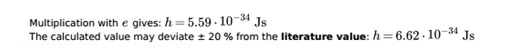Comparing to LD-DIDACTIC: The company's announced value is 7.9%.
After real measurement and discussed LD-Didactic technical staff, they also confirmed the actual error as follows:Using USB4000-UV-VIS spectroscopy (Oceanoptic - USA) to measure the spectrum of LEDs. We get the following results: